# 1445 Virgács for Your Boots Tonight

Tomorrow is Mikulás (Saint Nicholas Day) in Hungary. Children will awake to find candy, fruit, or nuts in their polished shoes or boots because every boy and every girl has been at least a little bit good all year long.

Because they have also been at least a little bit naughty, they will also find virgács in those same shoes or boots. Virgács are little twigs that have been spray-painted gold and tied together at the top with red ribbon.

Santa is so busy this time of year, that I thought I would give him a helping hand. I’ve made some virgács for YOUR boots or shoes!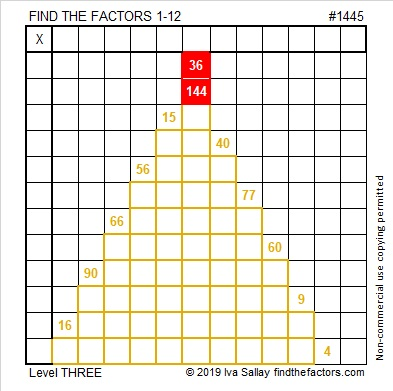Start at the top of the puzzle and work your way down cell by cell to solve this Level 3 puzzle. Oh, but I’ve been just a little bit naughty making this puzzle: you will need to look at later clues to figure out what factors to give to 40. Will clue 40 use a 5 or a 10? Look at clues 60 and 90, and you will have only one choice for that answer. Then you can forgive my tiny bit of naughtiness.

Now I’ll tell you a few facts about the puzzle number, 1445:

• 1445 is a composite number.
• Prime factorization: 1445 = 5 × 17 × 17, which can be written 1445 = 5 × 17²
• 1445 has at least one exponent greater than 1 in its prime factorization so √1445 can be simplified. Taking the factor pair from the factor pair table below with the largest square number factor, we get √1445 = (√289)(√5) = 17√5
• The exponents in the prime factorization are 1 and 2. Adding one to each exponent and multiplying we get (1 + 1)(2 + 1) = 2 × 3 = 6. Therefore 1445 has exactly 6 factors.
• The factors of 1445 are outlined with their factor pair partners in the graphic below.1445 is the sum of two squares in THREE different ways:
31² + 22² = 1445
34² + 17² = 1445
38² + 1² = 1445

1445 is the hypotenuse of SEVEN Pythagorean triples:
76-1443-1445 calculated from 2(38)(1), 38² – 1², 38² + 1²
221-1428-1445 which is 17 times (13-84-85)
477-1364-1445 calculated from 31² – 22², 2(31)(22), 31² + 22²
612-1309-1445 which is 17 times (36-77-85)
680-1275-1445 which is (8-15-17) times 85
805-1200-1445 which is 5 times (161-240-289)
867-1156-1445 which is (3-4-5) times 289 and can
also be calculated from 34² – 17², 2(34)(17), 34² + 17²

# 1444 Christmas Wrapping Paper

A fourth of the products in this multiplication table puzzle are already there just because I wanted the puzzle to have a wrapping paper pattern. Can you figure out what the factors are supposed to be and what all the other products are?• 1444 is a composite number and a perfect square.
• Prime factorization: 1444 = 2 × 2 × 19 × 19, which can be written 1444 = 2²× 19²
• 1444 has at least one exponent greater than 1 in its prime factorization so √1444 can be simplified. Taking the factor pair from the factor pair table below with the largest square number factor, we get √1444 = (√4)(√361) = (2)(19) = 38
• The exponents in the prime factorization are 1, 1, and 1. Adding one to each exponent and multiplying we get (2 + 1)(2 + 1) = 3 × 3 = 9. Therefore 1444 has exactly 9 factors.
• The factors of 1444 are outlined with their factor pair partners in the graphic below.Square number 1444 looks a lot like another square number, 144.
If we keep adding 4’s to the end, will we continue to get square numbers?
No.

However, in different bases, 1444 looks like several other square numbers:
It’s 484 in BASE 18,
400 in BASE 19,
169 in BASE 35,
144 in BASE 36,
121 in BASE 37, and
100 in BASE 38.

# 1443 A Simple Gift for You

This level one puzzle is my simple gift to you. Yes, you can solve it, and you don’t even have to wait until December 25th to discover all the factors and products to be found inside!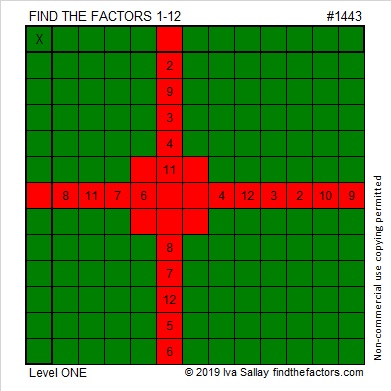That was puzzle number 1443. Here are some facts about that number.

• 1443 is a composite number.
• Prime factorization: 1443 = 3 × 13 × 37
• 1443 has no exponents greater than 1 in its prime factorization, so √1443 cannot be simplified.
• The exponents in the prime factorization are 1, 1, and 1. Adding one to each exponent and multiplying we get (1 + 1)(1 + 1)(1 + 1) = 2 × 2 × 2 = 8. Therefore 1443 has exactly 8 factors.
• The factors of 1443 are outlined with their factor pair partners in the graphic below.(38-1)(38+1) = 1443 so 1443 is one number away from 38² = 1444.

Actually, 1443 is the difference of two squares in four different ways:
38² – 1² = 1443
62²- 49² = 1443
242² – 239² = 1443
722² – 721² = 1443

1443 is the hypotenuse of FOUR Pythagorean triples:
93-1440-1443 which is 3 times (31-480-481)
468-1365-1443 which is (12-35-37) times 39
555-1332-1443 which is (5-12-13) times 111
957-1080-1443 which is 3 times(319-360-481)

# 1442 A Birthday Present for My Brother, Andy

My brother, Andy, has a birthday today. He’s very good at solving puzzles, so I made this Challenge puzzle as a  present for him.  Have a very happy birthday, Andy!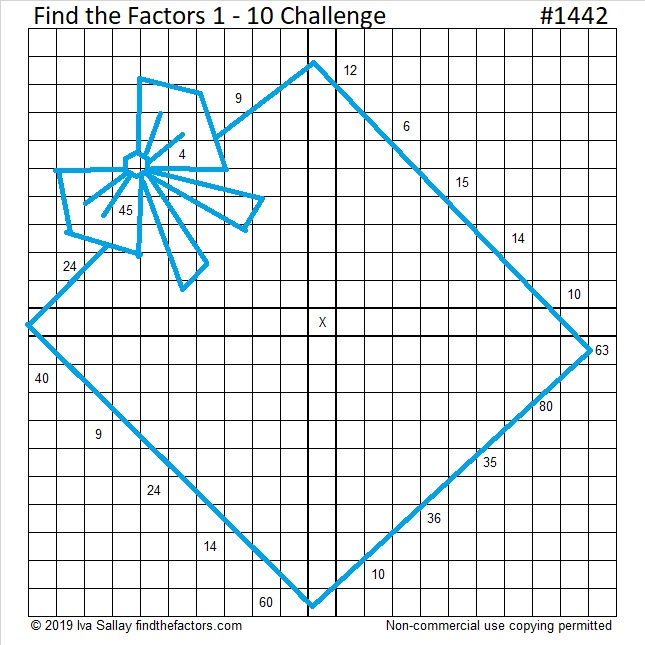You can try to solve it too. If the box and ribbon are too distracting, here’s a copy of the puzzle without the added color. Click on it to see it better.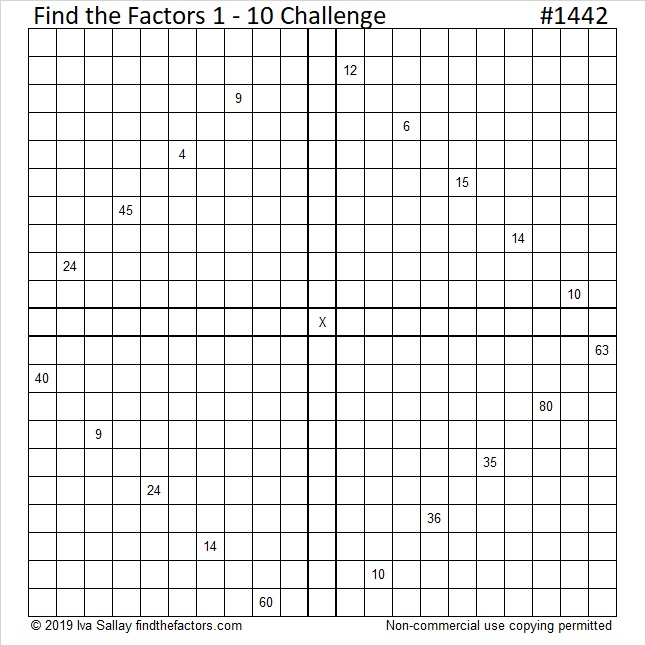Print the puzzles or type the solution in this excel file: 10 Factors 1432-1442

That was puzzle number 1442. Here are some facts about that number:

• 1442 is a composite number.
• Prime factorization: 1442 = 2 × 7 × 103
• 1442 has no exponents greater than 1 in its prime factorization, so √1442 cannot be simplified.
• The exponents in the prime factorization are 1, 1, and 1. Adding one to each exponent and multiplying we get (1 + 1)(1 + 1)(1 + 1) = 2 × 2 × 2 = 8. Therefore 1442 has exactly 8 factors.
• The factors of 1442 are outlined with their factor pair partners in the graphic below.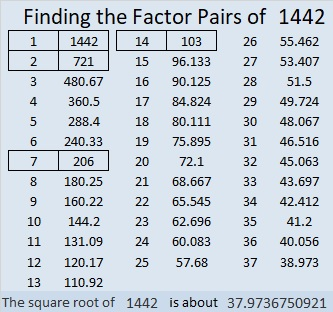2(103)(7) = 1442, making it a leg in this Pythagorean triple:
1442-10560-10658 calculated from 2(103)(7), 103² – 7², 103² + 7²

# Why is 1441 a Star?

1441 is the 16th star number. If you look at the tiny squares that make up each triangle in the star, you might notice that each of those triangles is made with 15 rows of squares. Thus each triangle represents the 15th triangular number.A six-pointed star is really just a 12-sided figure better known as a dodecagon.

Since I recently wrote that figurate numbers can be found using triangular numbers, I wondered if centered figurate numbers can make the same claim. Yes, they can!

Centered Figurate Numbers are also called Centered Polygonal Numbers and can be easily calculated from the triangular numbers.Here are some more facts about the number 1441:

• 1441 is a composite number.
• Prime factorization: 1441 = 11 × 131
• 1441 has no exponents greater than 1 in its prime factorization, so √1441 cannot be simplified.
• The exponents in the prime factorization are 1, and 1. Adding one to each exponent and multiplying we get (1 + 1)(1 + 1) = 2 × 2 = 4. Therefore 1441 has exactly 4 factors.
• The factors of 1441 are outlined with their factor pair partners in the graphic below.1441 is a palindrome with an even number of digits so it is divisible by 11.

1441 is also a palindrome in some other bases, namely, base 6, base 30, base 32, and base 36.

# Taking the Mystery out of 1440

The number 1440 has thirty-six factors. That’s a lot! The only number smaller than it with that many factors is 1260. Why do they have so many?

Would solving this Mystery Level puzzle be an easier mystery to solve?Print the puzzles or type the solution in this excel file: 10 Factors 1432-1442

• 1440 is a composite number.
• Prime factorization: 1440 = 2 × 2 × 2 × 2 × 2 × 3 × 3 × 5, which can be written 1440 = 2⁵ × 3² × 5
• 1440 has at least one exponent greater than 1 in its prime factorization so √1440 can be simplified. Taking the factor pair from the factor pair table below with the largest square number factor, we get √1440 = (√144)(√10) = 12√10
• The exponents in the prime factorization are 5,2, and 1. Adding one to each exponent and multiplying we get (5 + 1)(2 + 1)(1 + 1) = 6 × 3 × 2 = 36. Therefore 1440 has exactly 36 factors.
• The factors of 1440 are outlined with their factor pair partners in the graphic below.There you have it. The exponents of the prime factorization determine the number of factors a number has, and (5 + 1)(2 + 1)(1 + 1) = 6 × 3 × 2 = 36. How do we find its prime factorization? We can divide it over and over by the smallest prime number factor until we make an easy-to-read and very delicious cake!Or you could make a factor tree such as the one below. Then rake up the prime number factors afterward and hope that you see them all:I prefer cake to yard work any day!

As I mentioned before, 1440 is NOT the smallest number with 36 factors:
Because (2 + 1)(2 + 1)(1 + 1)(1 + 1) = 3 × 3 × 2 × 2 = 36 also, we get
2²× 3²× 5 × 7 = 1260, which just happens to be smaller than 1440, so 1260 gets the smallest-number-with-36-factors prize. 1440 is the SECOND smallest number with 36 factors.

1440 is the sum of the interior angles of a decagon. Why?
Because 180(10-2) = 180(8) = 1440
So what? 1260 is the sum of the interior angles of a nonagon.

1440 is the hypotenuse of only one Pythagorean triple:
864-1152-1440 which is (3-4-5) times 288
Yeah? 1260 is the hypotenuse of only one Pythagorean triple as well.

Look, 1440 is not second best because it has this one other claim to fame:
From Stetson.edu we learn that 1440 deserves a lot of exclamation points since
1440 = 2!3!5!

There you have it. We’ve taken the mystery out of the number 1440, AND it is a fabulous number!

# 1439 and Level 6

You know the factors of 49, but to complete this puzzle, you will also have to determine the answers to a few questions:

Will the common factor of 24 and 32 be 4 or 8?
Will the common factor of 20 and 40 be 4, 5, or 10?
Will the common factor of 27 and 9 be 3 or 9?

Don’t guess and check! Look at the other clues, and some of the possibilities will be eliminated*. Once you’ve found the next clue to use, continue using logic until the whole puzzle is completed.Print the puzzles or type the solution in this excel file: 10 Factors 1432-1442

• 1439 is a prime number.
• Prime factorization: 1439 is prime.
• 1439 has no exponents greater than 1 in its prime factorization, so √1439 cannot be simplified.
• The exponent in the prime factorization is 1. Adding one to that exponent we get (1 + 1) = 2. Therefore 1439 has exactly 2 factors.
• The factors of 1439 are outlined with their factor pair partners in the graphic below.How do we know that 1439 is a prime number? If 1439 were not a prime number, then it would be divisible by at least one prime number less than or equal to √1439. Since 1439 cannot be divided evenly by 2, 3, 5, 7, 11, 13, 17, 19, 23, 29 31, or 37, we know that 1439 is a prime number.

* Did you notice that either one of the 24’s or 6 must use a 3 because only two 6s are allowed to be used as factors? What does that tell you about the common factor of 27 and 9?

# 1438 and Level 5

You can solve this puzzle by using logic and multiplication/division facts. The unique solution requires all the numbers from 1 to 10 in both the first column and the top row. Can you solve it?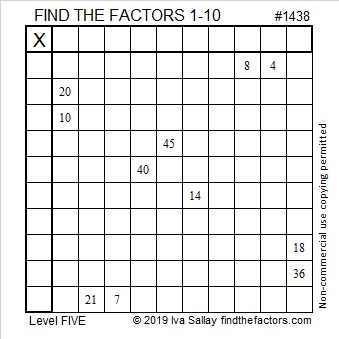Print the puzzles or type the solution in this excel file:  10 Factors 1432-1442

Today’s puzzle was given the number 1438. Here are a few facts about that number:

• 1438 is a composite number.
• Prime factorization: 1438 = 2 × 719
• 1438 has no exponents greater than 1 in its prime factorization, so √1438 cannot be simplified.
• The exponents in the prime factorization are 1, and 1. Adding one to each exponent and multiplying we get (1 + 1)(1 + 1) = 2 × 2 = 4. Therefore 1438 has exactly 4 factors.
• The factors of 1438 are outlined with their factor pair partners in the graphic below.1438 is the sum of four consecutive numbers:
358  + 359 + 360 + 361 = 1438

# 1437 Belt Buckle Mystery

I have childhood memories of preparing for Thanksgiving at school by making pilgrim hats, pilgrim shirts, or pilgrim shoes out of construction paper. Each of those clothing items had a distinguished buckle. Why? That buckle’s popularity was a mystery to me until I read an article by Ken Jennings titled The Debunker: What Did Pilgrim Hats Really Look Like?

This mystery level puzzle has a buckle, too. Perhaps it can help debunk the idea that math is no fun while it helps you learn about factors or reinforces your memory of the multiplication table.Print the puzzles or type the solution in this excel file:  10 Factors 1432-1442

The puzzle number is 1437. Here are a few facts about that number:

• 1437 is a composite number.
• Prime factorization: 1437 = 3 × 479
• 1437 has no exponents greater than 1 in its prime factorization, so √1437 cannot be simplified.
• The exponents in the prime factorization are 1, and 1. Adding one to each exponent and multiplying we get (1 + 1)(1 + 1) = 2 × 2 = 4. Therefore 1437 has exactly 4 factors.
• The factors of 1437 are outlined with their factor pair partners in the graphic below.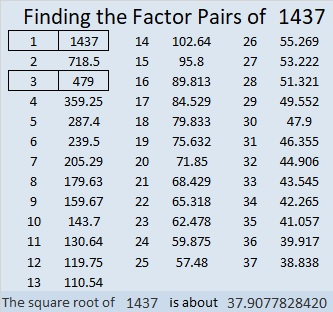1437 is the difference of two squares in two different ways:
719² – 718² = 1437
241² – 238² = 1437

# 1436 This Turkey Is Ready

If this year you find yourself needing a bigger turkey, then this one fits that description. This turkey uses math facts from a 14 × 14 multiplication table.

Adding prime number 13 doesn’t cause any problems. You hopefully will recognize that 39 and 119 are both multiples of 13.

However, adding 14 to the puzzle might make you want to call “fowl” because clues 28, 56, 70, and 84 all have two sets of factor pairs where both factors are 14 or less. Can you figure out which clues use 7 and which use 14?

Like always, there is only one solution.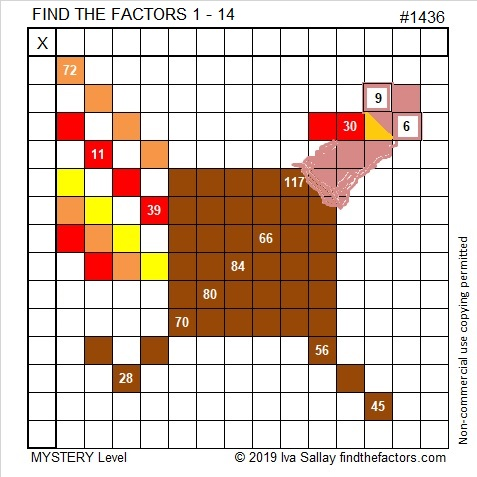Print the puzzles or type the solution in this excel file:  10 Factors 1432-1442

I enjoyed this tweet from Robin Schwartz. Spoiler alert: If you click on the link, you will see the solution.

That was puzzle number 1436. Here are a few facts about that number:

• 1436 is a composite number.
• Prime factorization: 1436 = 2 × 2 × 359, which can be written 1436 = 2² × 359
• 1436 has at least one exponent greater than 1 in its prime factorization so √1436 can be simplified. Taking the factor pair from the factor pair table below with the largest square number factor, we get √1436 = (√4)(√359) = 2√359
• The exponents in the prime factorization are 2 and 1. Adding one to each exponent and multiplying we get (2 + 1)(1 + 1) = 3 × 2 = 6. Therefore 1436 has exactly 6 factors.
• The factors of 1436 are outlined with their factor pair partners in the graphic below.1436 is the difference of two squares:
360² – 358² = 1436